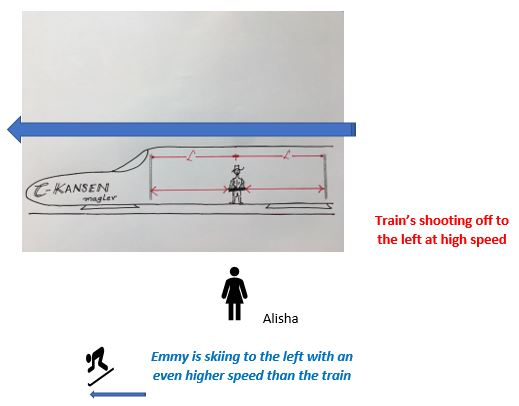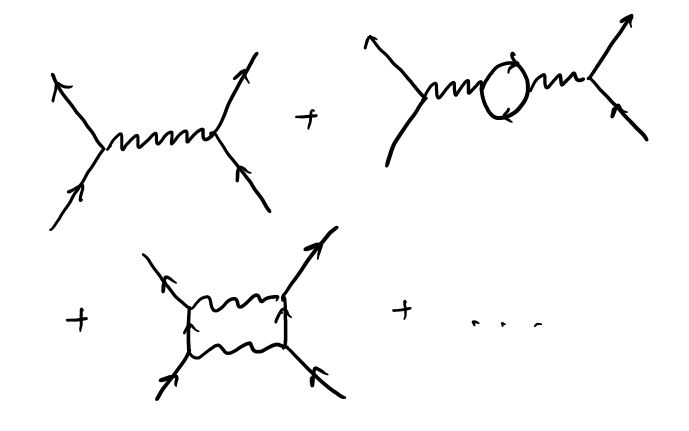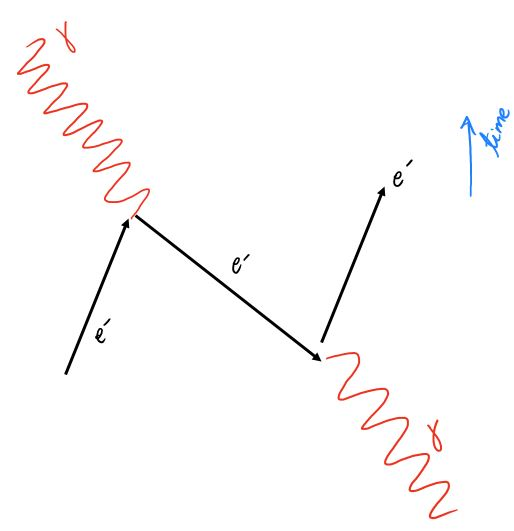# Special Relativity; Or how I learned to relax and love the Anti-ParticleThe Special Theory of Relativity, which is the name for the set of ideas that Einstein proposed in 1905 in a paper titled “On the Electrodynamics of moving bodies”, starts with the premise that the Laws of Physics are the same for all observers that are traveling at uniform speeds relative to each other. One of the Laws of Physics includes a special velocity – Maxwell’s equations for electromagnetism include a special speed$c$, which is the same for all observers. This leads to some spectacular consequences. One of them is called the “Relativity of Simultaneity”. Let’s discuss this with the help of the picture below.Babu is sitting in a railway carriage, manufactured by the famous C-Kansen company, that travels at speeds close to that of light. Babu’s sitting exactly in the middle of the carriage and for reasons best known to himself (I guess the pantry car was closed and he was bored), decides to shoot a laser beam simultaneously at either end of the carriage from his position. There are detectors/mirrors that detect the light at the two ends of the carriage. As far as he is concerned, light travels at$3 \times 10^5 \frac {km}{sec}$ and he will challenge anyone who says otherwise to a gunfight – note that he is wearing a cowboy hat and probably practices open carry.

Since the detectors at the end of the carriage are equidistant from him, he is going to be sure to find the laser beams hit the detectors simultaneously, from his point of view.

Now, consider the situation from the point of view of Alisha, standing outside the train, near the tracks, but safely away from Babu and his openly carried munitions. She sees that the train is speeding away to the left, so clearly since${\bf she}$ thinks light also travels at$3 \times 10^5 \frac {km}{sec}$, she would say that the light hit the${\bf right}$ detector first before the${\bf left}$ detector. She doesn’t${\underline {at \: all \: think}}$ that the light hit the two detectors simultaneously. If you asked her to explain, she’d say that the right detector is speeding towards the light, while the left detector is speeding away from the light, which is why the light strikes them at different times.

Wait – it is worse. If you had a third observer, Emmy, who is skiing to the${\bf {left}}$ at an even higher speed than the C-Kansen (some of these skiers are crazy), she thinks the C-Kansen train is going off to the right (think about it), not able to keep up with her. As far as${\underline {\bf {she}}}$ is concerned, the laser beam hit the${\bf {left}}$ detector before the other beam hit the${\bf {right}}$ detector.

What are we finding? The Events in question are – “Light hits Left Detector” and “Light hits Right Detector”. Babu claims the two events are simultaneous. Alisha claims the second happened earlier. Emmy is insistent that the first happened earlier. Who is right?

They are ALL correct, in their own reference frames. Events that appear simultaneous in one reference frame can appear to occur the one before the other in a different frame, or indeed the one after the other, in another frame. This is called the Relativity of Simultaneity. Basically, this means that you cannot attribute one of these events to have${\bf {caused}}$ the other, since their order can be changed. Events that are separated in this fashion are called “space-like separated”.

Now, on to the topic of this post. In the physics of quantum field theory, particles interact with each other by exchanging other particles, called gauge bosons. This interaction is depicted, in very simplified fashion so we can calculate things like the effective force between the particles, in a sequence of diagrams called Feynman diagrams. Here’s a diagram that depicts the simplest possible interaction between two electronsTime goes from the bottom to the top, the electrons approach each other, exchange a photon, then scoot off in different directions.

This is the simplest diagram, though and to get the exact numerical results for such scattering, you have to add higher orders of this diagram, as shown belowWhen you study such processes, you have to perform mathematical integrals – all you know is that you sent in some particles from far away into your experimental set-up, something happened and some particles emerged from inside. Since you don’t know where and when the interaction occurred (where a particle was emitted or picked up, as at the vertexes in the above diagrams), you have to sum over all possible places and times that the interaction${\bf {could}}$ have occurred.

Now comes the strange bit. Look at what might happen when you sum over all possible paths for a collision between an electron and a photon.In the above diagram, the exchange was simultaneous.

In the next one, the electron emitted a photon, then went on to absorb a photon.and then comes the strange bit –Here the electron emitted a photon, then went backwards in time, absorbed a photon, then went its way.

When we sum over all possible event times and locations, this is really what the integrals in quantum field theory instruct us to do!

Really, should we allow ourselves to count processes where  two events occur simultaneously, which means we would then have to allow for them to happen in reverse order, as in the third diagram? What’s going on? This has to be wrong! And what’s an electron going backwards in time anyway? Have we ever seen such a thing?

Could we simply ban such processes? So, we would only sum over positions and times where the intermediate particles had enough time to go from one place to another,

There’s a problem with this. Notice the individual vertexes where an electron comes in, emits (or absorbs) a photon, then moves on. If this were a “real” process, it wouldn’t be allowed. It violates the principle of energy-momentum conservation. A simple way to understand this is to ask, could a stationary electron suddenly emit a photon and shoot off in a direction opposite to the photon, It looks deceptively possible! The photon would have, say, energy$E$ and momentum$p = E/c$. This means that the electron would also have momentum$E/c$, in the opposite direction (for conservation) but then its energy would have to be$\sqrt{E^2+m^2 c^4}$ from the relativistic formula. This is higher than the energy$m c^2$ of the initial electron :$A+ \sqrt{A^2+m^2}$ is bigger than$m$! Not allowed !

We are stuck. We have to assume that energy-momentum conservation is violated in the intermediate state – in all possible ways. But then, all hell breaks loose – in relativity, the speed of a particle$v$ is related to its momentum$p$ and its energy$E$ by$v = \frac {p}{E}$ – since$p$ and$E$ can be${\underline {anything}}$, the intermediate electron could, for instance, travel faster than light. If so, in the appropriate reference frame, it would be absorbed before it was created. If you can travel faster than light, you can travel backwards in time (read this post in Matt Buckley’s blog for a neat explanation).

If the electron were uncharged, we would probably be hard-pressed to notice. But the electron is charged. This means if we had the following sequence of events,

– the world has -1 net charge

– electron emits a photon and travels forward in time

– electron absorbs a photon and goes on.

This sequence doesn’t appear to change the net charge in the universe.

But consider the following sequence of events

– the world has -1 net charge

– the electron emits a photon and travels backwards in time

– the electron absorbs a photon in the past and then starts going forwards in time

Now, at some intermediate time, the universe seems to have developed two extra negative charges.

This can’t happen – we’d notice! Extra charges tend to cause trouble, as you’d realize if you ever received an electric shock.

The only way to solve this is to postulate that an electron moving backward in time has a positive charge. Then the net charge added for all time slices is always -1.

ergo, we have antiparticles. We need to introduce the concept to marry relativity with quantum field theory.

There is a way out of this morass if we insist that all interactions occur at the same point in space and that we never have to deal with “virtual” particles that violate momentum-energy conservation at intermediate times. This doesn’t work because of something that Ken Wilson discovered in the late ’70s, called the renormalization group – the results of our insistence would be we would disagree with experiment – the effects would be too weak.

For quantum-field-theory students, this is basically saying that the expansion of the electron’s field operator into its components can’t simply be$\Psi(\vec x, t) = \sum\limits_{spin \: s} \int \frac {d \vec k}{(2 \pi)^3} \frac{1}{\sqrt{2 E_k}} b_s(\vec k) e^{- i E_k t + i {\vec k}.{\vec x}}$

but has to be$\Psi(\vec x, t) = \sum\limits_{spin \: s} \int \frac {d \vec k}{(2 \pi)^3} \frac{1}{\sqrt{2 E_k}} \left( b_s(\vec k) e^{- i E_k t + i {\vec k}.{\vec x}} +d^{\dagger}_s(\vec k) e^{+ i E_k t - i {\vec k}.{\vec x}} \right)$

including particles being destroyed on par with anti-particles being created and vice versa.

The next post in this sequence will discuss another interesting principle that governs particle interactions – C-P-T.

Quantum field theory is an over-arching theory of fundamental interactions. One bedrock of the theory is something called C-P-T invariance.  This means that if you take any physical situation involving any bunch of particles, then do the following

• make time go backwards
• parity-reverse space (so in three-dimensions, go into a mirror world, where you and everything else is opposite-handed)
• change all particles into anti-particles (with the opposite charge)

then you will get a process which could (and should) happen in your own world. As far as we know this is always right, in general and it has been proven under a variety of assumptions. A violation of the C-P-T theorem in the universe would create quite a stir. I’ll discuss that in a future post.

Addendum: After this article was published, I got a message from someone I respect a huge amount that there is an interesting issue here. When we take a non-relativistic limit of the relativistic field theory, where do the anti-particles vanish off to? This is a question that is I am going to try and write about in a bit!

1.Skanda Rao says:

How literally could we take the interpretation of anti particles as particles moving backwards in time? For instance, if we could somehow make stable anti matter systems, would entropy decrease over time and increase in reverse time as long as these interactions are isolated from normal matter? Do antimatter glass shards rise up from the floor to form a wine glass? If CPT invariance as you briefly described it is true, does it suggest a sort of reverse causality where antimatter from the future could have an effect on matter now through QFT interactions?
A little more fundamental: what does a direction in time mean? Motion in space is usually described with changes over time as time progresses in a forward direction – otherwise, without another dimension as reference, motion in space is described with a path with no definite direction. What is the distinction, if any, between moving backwards in time and moving forwards in time in the other direction in space, switching the labeling of the creation and annihilation of a particle in space time? I’d be especially confused about that if anti-particles actually exhibited reverse entropy.

1.Simply Curious says:

There are many questions here – I will address a couple.

First, both particles and anti-particles have positive mass. Dirac’s initial interpretation of anti-particles was made in order to eliminate the possibility that all particles would simply jump to negative energy states, thus eliminating the universe in a puff of radiation. He posited that the negative energy states were filled and an excitation from the negative energy state creates two entities – a positive energy particle and a positive energy object that is the absence of a negative energy state ( a hole ). You might know of holes from semi-conductor physics – they are positive energy states, which is why your radio or TV doesn’t collapse into a puff of nothingness either.

In addition, gravity is described by a spin-2 field theory and in such a theory, like-charges attract. Its a consequence of math (maybe good for a future post). The same math also explains why, in a spin-1 theory (the theory of the electromagnetic field), like charges repel. This, to be sure, doesn’t “explain” anything in any more fundamental way – the structure of our theories is consistent with nature. So, matter and anti-matter will still attract each other.

There is a deep reason why there are only spin-0,1/2,1,3/2,2 fundamental particles in nature (though it is not really clear why we haven’t seen a spin 3/2 particle yet). It arises, again, from special relativity and Lorentz invariance as well as fundamental principles of quantum field theory – maybe a good topic for yet another post!

I have to think about a careful answer to your entropy question. But keep coming on with the questions!

This blog post might be interesting reading..
http://motls.blogspot.cz/2010/09/can-antimatters-gravity-be-repulsive.html?m=1

2.Skanda Rao says:

And the questions keep coming – what about gravitational fields? Don’t straight lines in a curved space time imply that in reverse time, anti-particles are repelled by large, massive objects? Is it symmetric – would we be repelled by massive antimatter objects?
If an anti-atom created in our labs fell into a black hole, would the reversed view be that the black hole created an atom which ended up being destroyed in a physics lab?
What if it was a stable antimatter crystal – would the black hole have created a complex crystalline structure in reverse time?
I should also mention that I love the title for this article.